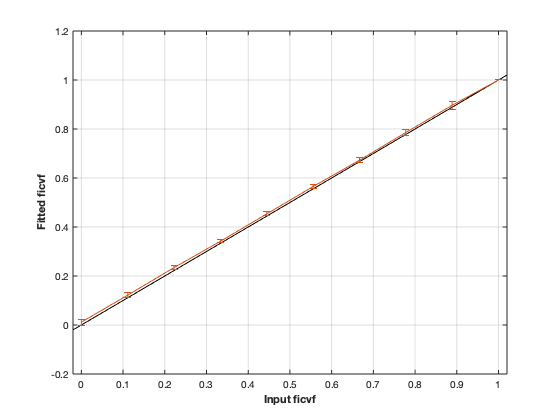# noddi: Neurite Orientation Dispersion and Density Imaging¶## Contents

```% This m-file has been automatically generated using qMRgenBatch(noddi)
% Command Line Interface (CLI) is well-suited for automatization
% purposes and Octave.
%
% Please execute this m-file section by section to get familiar with batch
% processing for noddi on CLI.
%
%
% Written by: Agah Karakuzu, 2017
% =========================================================================
```

## I- DESCRIPTION

```qMRinfo('noddi'); % Describe the model
```
```  noddi:   Neurite Orientation Dispersion and Density Imaging
Three-compartment model for fitting multi-shell DWI
a href="matlab: figure, imshow Diffusion.png ;"Pulse Sequence Diagram/a

ASSUMPTIONS:
Neuronal fibers model:
geometry                          sticks (Dperp = 0)
Orientation dispersion            YES (Watson distribution). Note that NODDI is more robust to
crossing fibers that DTI  (Campbell, NIMG 2017)

Permeability                      NO
Diffusion properties:
intra-axonal                      totally restricted
diffusion coefficient (Dr)      fixed by default.
extra-axonal                      Tortuosity model. Parallel diffusivity is equal to
intra-diffusivity.Perpendicular diffusivity is
proportional to fiber density
diffusion coefficient (Dh)      Constant

Inputs:
DiffusionData       4D diffusion weighted dataset

Outputs:
di                  Diffusion coefficient in the restricted compartment.
ficvf               Fraction of water in the restricted compartment.
fiso                Fraction of water in the isotropic compartment (e.g. CSF/Veins)
fr                  Fraction of restricted water in the entire voxel (e.g. intra-cellular volume fraction)
fr = ficvf*(1-fiso)
diso (fixed)        diffusion coefficient of the isotropic compartment (CSF)
kappa               Orientation dispersion index
b0                  Signal at b=0
theta               angle of the fibers
phi                 angle of the fibers

Protocol:
Multi-shell diffusion-weighted acquisition
at least 2 non-zeros bvalues
at least 5 b=0 (used to compute noise standard deviation

DiffusionData       Array [NbVol x 7]
Delta (s)         Diffusion separation
delta (s)         Diffusion duration
TE (s)            Echo time

Options:
Model               Model part of NODDI.
Available models are:
-WatsonSHStickTortIsoVIsoDot_B0 is a four model compartment used for ex-vivo datasets

Example of command line usage
For more examples: a href="matlab: qMRusage(noddi);"qMRusage(noddi)/a

Author: Tanguy Duval

References:
Please cite the following if you use this module:
Zhang, H., Schneider, T., Wheeler-Kingshott, C.A., Alexander, D.C., 2012. NODDI: practical in vivo neurite orientation dispersion and density imaging of the human brain. Neuroimage 61, 1000?1016.
In addition to citing the package:
Karakuzu A., Boudreau M., Duval T.,Boshkovski T., Leppert I.R., Cabana J.F.,
Gagnon I., Beliveau P., Pike G.B., Cohen-Adad J., Stikov N. (2020), qMRLab:
Quantitative MRI analysis, under one umbrella doi: 10.21105/joss.02343

Reference page in Doc Center
doc noddi

```

## a- create object

```Model = noddi;
```

## b- modify options

```         |- This section will pop-up the options GUI. Close window to continue.
|- Octave is not GUI compatible. Modify Model.options directly.```
```Model = Custom_OptionsGUI(Model); % You need to close GUI to move on.
```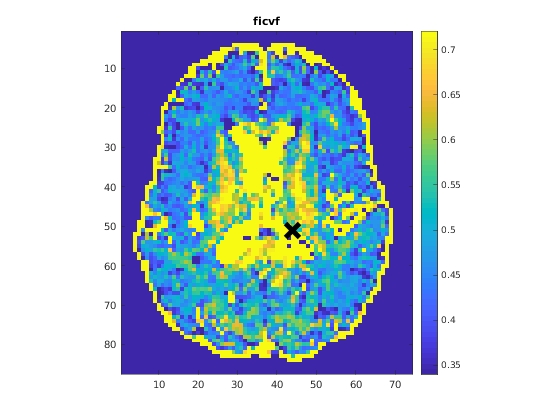## III- FIT EXPERIMENTAL DATASET

```         |- noddi object needs 2 data input(s) to be assigned:
|-   DiffusionData
```data = struct();
% DiffusionData.nii.gz contains [74   87   50  109] data.
% Mask.nii.gz contains [74  87  50] data.
```

## b- fit dataset

`           |- This section will fit data.`
```FitResults = FitData(data,Model,0);
```
```=============== qMRLab::Fit ======================
Operation has been started: noddi
Elapsed time is 1.845969 seconds.
Operation has been completed: noddi
==================================================
```

## c- show fitting results

```         |- Output map will be displayed.
|- If available, a graph will be displayed to show fitting in a voxel.
|- To make documentation generation and our CI tests faster for this model,
we used a subportion of the data (40X40X40) in our testing environment.
|- Therefore, this example will use FitResults that comes with OSF data for display purposes.
|- Users will get the whole dataset (384X336X224) and the script that uses it for demo
via qMRgenBatch(qsm_sb) command.```
```FitResults_old = load('FitResults/FitResults.mat');
qMRshowOutput(FitResults_old,data,Model);
```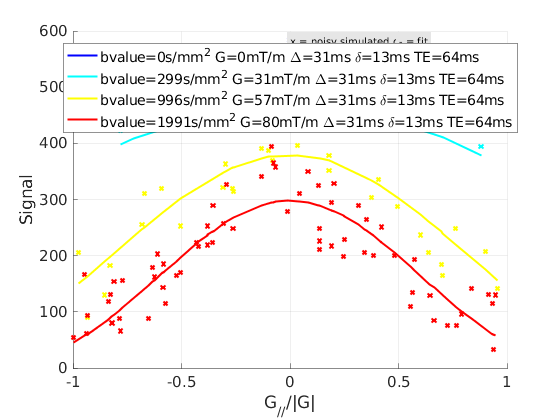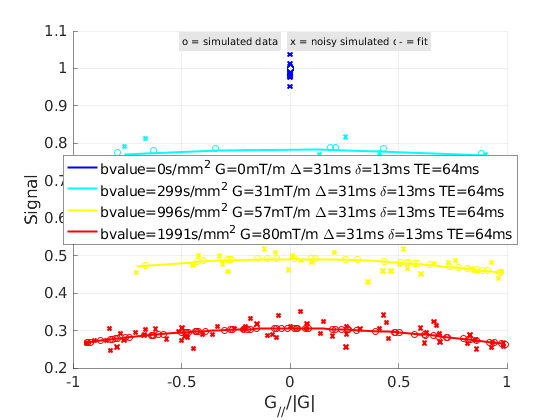## d- Save results

```         |-  qMR maps are saved in NIFTI and in a structure FitResults.mat
that can be loaded in qMRLab graphical user interface
|-  Model object stores all the options and protocol.
It can be easily shared with collaborators to fit their
own data or can be used for simulation.```
```FitResultsSave_nii(FitResults, 'noddi_data/DiffusionData.nii.gz');
Model.saveObj('noddi_Demo.qmrlab.mat');
```
```Warning: Directory already exists.
```

## V- SIMULATIONS

`   |- This section can be executed to run simulations for noddi.`

## a- Single Voxel Curve

```         |- Simulates Single Voxel curves:
(1) use equation to generate synthetic MRI data
(3) fit and plot curve```
```      x = struct;
x.ficvf = 0.5;
x.di = 1.7;
x.kappa = 0.05;
x.fiso = 0;
x.diso = 3;
x.b0 = 1;
x.theta = 0.2;
x.phi = 0;
Opt.SNR = 50;
% run simulation
figure('Name','Single Voxel Curve Simulation');
FitResult = Model.Sim_Single_Voxel_Curve(x,Opt);
```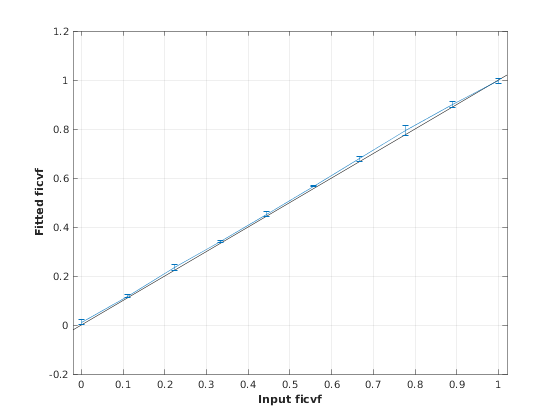## b- Sensitivity Analysis

```         |-    Simulates sensitivity to fitted parameters:
(1) vary fitting parameters from lower (lb) to upper (ub) bound.
(2) run Sim_Single_Voxel_Curve Nofruns times
(3) Compute mean and std across runs```
```      %              ficvf         di            kappa         fiso          diso          b0            theta         phi
OptTable.st = [0.5           1.7           0.05          0             3             1             0.2           0]; % nominal values
OptTable.fx = [0             1             1             1             1             1             1             1]; %vary ficvf...
OptTable.lb = [0             1.3           0.05          0             1             0             0             0]; %...from 0
OptTable.ub = [1             2.1           0.8           1             5             1e+03         3.1           3.1]; %...to 1
Opt.SNR = 50;
Opt.Nofrun = 5;
% run simulation
SimResults = Model.Sim_Sensitivity_Analysis(OptTable,Opt);
figure('Name','Sensitivity Analysis');
SimVaryPlot(SimResults, 'ficvf' ,'ficvf' );
```